# Calculating Interest Worksheets

#### How to Calculate Simple Interest

The expression "basic" signifies you are working with the easiest method of computing interest. When you see how to compute a straightforward premium, you can proceed onward to different computations, such as yearly rate yield (APY), yearly rate (APR), and progressive accrual. Let us assume you contribute \$100 (the head) at a 5% yearly rate for one year. The straightforward interest figuring is: \$100 x 0.05 x 1 = \$5 straightforward interest for one year Note that the loan cost (5%) shows up as a decimal (.05). To do your own calculations, you may need to change rates over to decimals. A simple stunt for recalling this is to consider the word percent "per 100." You can change over a rate into its decimal structure by partitioning it by 100. Or on the other hand, simply move the decimal direct two spaces toward the left. For instance, to change over 5% into a decimal, partition 5 by 100 and get .05.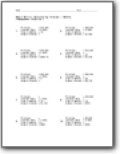###### (Months) - Basic Skills

We provide you with four variables (principal, interest rate, time in months, and simple interest accrued), one is missing. What is that value?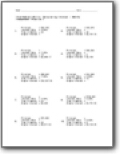###### (Months) - Intermediate Skills

We work with large values in this series.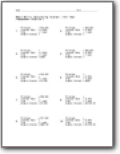###### Full Year

We pivot from using the time frame of a few months to a single year.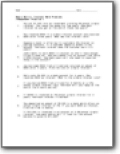###### Basic Skills: Interest Word Problems

Example problem: You put \$1,000 into an investment yielding 6% annual simple interest. You leave the money for two years. How much interest do you get at the end of those two years?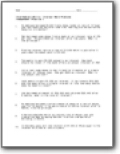###### Intermediate Skills: Interest Word Problems

Example problem: An employee borrowed \$5,000 from a money lender at rate of 12 % per year and repaid \$5,450 after some time. How long did she borrow the money for (in months)?